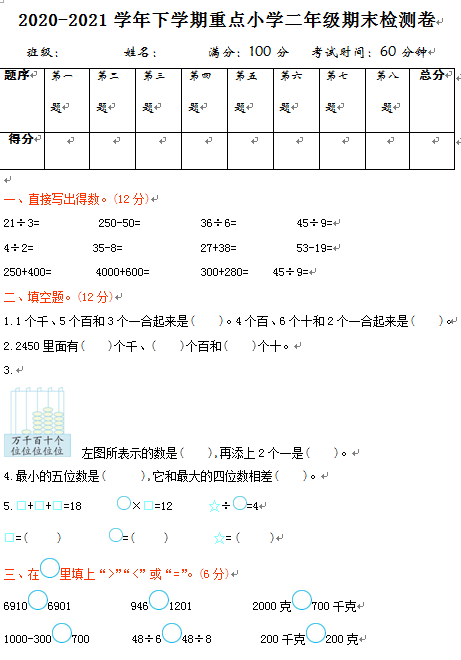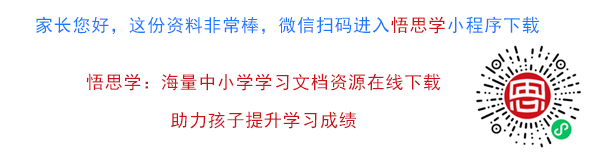2020-2021学年下学期重点小学二年级期末检测卷

21÷3=　　　　　250-50=　　　　　36÷6=　　　　　45÷9=

4÷2=            35-8=              27+38=             53-19=

250+400=          4000+600=            300+280=     45÷9=

1.1个千、5个百和3个一合起来是(　　)。4个百、6个十和2个一合起来是(　　)。

2.2450里面有(　　)个千、(　　)个百和(　　)个十。

【DOC文档6页】2020-2021学年下学期重点小学二年级期末数学检测卷（含答案）A4电子版资料_可直接打印_会员免费下载##### 评论信息